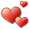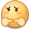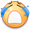# 雅乐网

## Modularity的计算方法——社团检测中模块度计算公式详解

Modularity，中文称为模块度，是 Community Detection（社区发现/社团检测） 中用来衡量社区划分质量的一种方法。要理解Modularity，我们先来看社团和社团检测的概念。

### 社团检测### 公式中用到的字母

#### 和图相关的

n 表示图的结点个数，这里n=14（结点编号0到13）

m 表示图中边的个数，这里m=23（上图中共23条边）

k 表示图中结点的度（degree），无向图中一个结点的度就是该结点连出去的边的条数。显然图中14个结点都有自己的度，我们用$$k_i$$来表示结点i的度。例如：$$k_0 = 3, k_5 = 4, k_6 = 2$$。

A 表示图的邻接矩阵。邻接矩阵A，$$A_{ij} = 1$$表示 结点v 和 结点w 之间有一条边， $$A_{ij} = 0$$表示 结点i 和 结点j 之间没有边。无向图的邻接矩阵是对称的，即$$A_{ij} = A_{ji}$$。特别的，这里结点自己和自己的连接是0，即$$A_{ii} = 0$$，不考虑结点自己和自己的连边。

### Modularity第一版

Newman 在2003年的论文 “Finding and evaluating community structure in networks” 中首次提出了modularity的定义，在论文中用来度量自己的社团检测算法的好坏。

Consider a particular division of a network into k communities. Let us define a k×k symmetric matrix e whose element $$e_{ij}$$ is the fraction of all edges in the network that link vertices in community i to vertices in community j .As discussed in , it is crucial to make sure each edge is counted only once in the matrix eij—the same edge should not appear both above and below the diagonal. Alternatively, an edge linking communities i and j can be split, half-and-half, between the ij and ji elements, which has the advantage of making the matrix symmetric. Either way, there are a number of factors of 2 in the calculation that must be watched carefully, lest they escape one’s attention and make mischief.

$$e_{32} = \frac{2}{23}， e_{23} = \frac{0}{23}$$ 或者 $$e_{32} = \frac{0}{23}， e_{23} = \frac{2}{23}$$ 或者$$e_{32} = \frac{1}{23} ， e_{23} = \frac{1}{23}$$

 1 2 3 1 $$\frac{5}{23}$$ $$\frac{1}{2} \times \frac{1}{23}$$ $$\frac{1}{2} \times \frac{0}{23}$$ 2 $$\frac{1}{2} \times \frac{1}{23}$$ $$\frac{7}{23}$$ $$\frac{1}{2} \times \frac{2}{23}$$ 3 $$\frac{1}{2} \times \frac{0}{23}$$ $$\frac{1}{2} \times \frac{2}{23}$$ $$\frac{8}{23}$$

So we further define the row (or column) sums $$a_i = \sum_{j}{e_{ij}}$$ , which represent the fraction of edges that connect to vertices in community i.

$$a_i = \sum_{j}{e_{ij}}$$，它表示连接到 社团i 中的边的端点数（相当于社团中所有点的度相加） 占总端点数（2m） 的比例 ，即

$$a_i = \frac{k_{C_i}}{2m}$$

$$k_{C_i}$$表示社团i内部所有点的度数之和。

 a 1 $$\frac{5}{23} + \frac{1}{2} \times \frac{1}{23} + \frac{1}{2} \times \frac{0}{23}$$ = $$\frac{11}{46}$$ 2 $$\frac{1}{2} \times \frac{1}{23} + \frac{7}{23} + \frac{1}{2} \times \frac{2}{23}$$ = $$\frac{17}{46}$$ 3 $$\frac{1}{2} \times \frac{0}{23} + \frac{1}{2} \times \frac{2}{23} + \frac{8}{23}$$ = $$\frac{18}{46}$$

In a network in which edges fall between vertices without regard for the communities they belong to, we would have $$e_{ij} = a_ia_j$$.

Thus we can define a modularity measure by

$$Q = \sum_i{(e_{ii} – a_i^2)} = Tr e – ||e^2||$$

where ||x|| indicates the sum of the elements of the matrix x.

$$Q = \sum_i{(e_{ii} – a_i^2)} = \sum_i{e_{ii}} – \sum_i{ a_i^2}$$

$$Q = \sum_i{(\frac{e_i}{m} – (\frac{k_{C_i}}{2m})^2)}$$

$$Q = \frac{5}{23} + \frac{7}{23} + \frac{8}{23} – (\frac{11}{46})^2 – (\frac{17}{46})^2 – (\frac{18}{46})^2 = \frac{553}{1058} = 0.52268431$$

$$Q = \sum_i{(e_{ii} – a_i^2)} = \sum_i{e_{ii}} – \sum_i{ a_i^2} = Tr e – ||e^2||$$

$$\sum_i{ ( \sum_{j}{e_{ij}} )^2} = ||e^2||$$

$$\sum_i{ a_i^2} = (\frac{5}{23} + \frac{1}{46} + 0)^2 + (\frac{1}{46} + \frac{7}{23} + \frac{1}{23})^2 + (0 + \frac{1}{23} + \frac{8}{23})^2$$

$$e^2 = \begin{pmatrix} \frac{5}{23} & \frac{1}{46} & 0 \\ \frac{1}{46} & \frac{7}{23} & \frac{1}{23} \\ 0 & \frac{1}{23} & \frac{8}{23} \\ \end{pmatrix} \times \begin{pmatrix} \frac{5}{23} & \frac{1}{46} & 0 \\ \frac{1}{46} & \frac{7}{23} & \frac{1}{23} \\ 0 & \frac{1}{23} & \frac{8}{23} \\ \end{pmatrix} =$$

### Modularity第二版

#### Newman论文中的定义

2006年，Newman 在论文“Modularity and community structure in networks”中重新定义了modularity，目的是为了满足spectral properties。原话为：

Here we take a different approach based on a reformulation of the modularity in terms of the spectral properties of the network of interest.

Suppose our network contains n vertices.

n 表示图的结点个数

For a particular division of the network into two groups let s_i = 1 if vertex i belongs to group 1 and s_i = -1 if it belongs to group 2. Observing that the quantity $$\frac{1}{2} (s_i s_j + 1)$$  is 1 if i and j are in the same group and 0 otherwise

And let the number of edges between vertices i and j be $$A_{ij}$$, which will normally be 0 or 1, although larger values are possible in networks where multiple edges are allowed.(The quantities $$A_{ij}$$ are the elements of the so-called adjacency matrix.)

1. 无向图的邻接矩阵是对称的，即$$A_{ij} = A_{ji}$$。因此，如果把矩阵A的所有元素相加，得到的值是图中边的数目的2倍：$$2m = \sum{A_{ij}}$$。

2. 自己和自己的连接在矩阵中是0，即$$A_{ii} = 0$$。

At the same time, the expected number of edges between vertices i and j if edges are placed at random is $$\frac{k_ik_j}{2m}$$, where $$k_i$$ and $$k_j$$ are the degrees of the vertices and $$m = \frac{1}{2} \sum_i{k_i}$$ is the total number of edges in the network.

Thus the modularity Q is given by the sum of $$A_{ij} – \frac{k_ik_j}{2m}$$   over all pairs of vertices i, j that fall in the same group.

$$A_{ij} – \frac{k_ik_j}{2m}$$ 表示的是结点i和结点j之间的连边数，减去 随机情况下 结点i和结点j之间的期望连边数。（关于随机情况的讨论在下面）

we can then express the modularity as

$$Q = \frac{1}{4m} \sum_{ij}{(A_{ij} – \frac{k_ik_j}{2m})(s_is_j+1)}$$

The leading factor of $$\frac{1}{4m}$$ is merely conventional: it is included for compatibility with the previous definition of modularity (17).

Q计算公式前面的$$\frac{1}{4m}$$仅仅是为了和之前第一版的modularity计算公式相兼容。

$$Q = \frac{1}{2m} \sum_{ij}{(A_{ij} – \frac{k_ik_j}{2m})\frac{(s_is_j+1)}{2}}$$

$$Q = \frac{1}{2m} \sum_{ij}{(A_{ij} – \frac{k_ik_j}{2m}) \delta(i, j)}$$

$$Q = \frac{1}{2m} \sum_{i,j在同一社团}{(A_{ij} – \frac{k_ik_j}{2m}) }$$

$$A_{ij}$$ 表示 结点i 和 结点j 之间边的数目

$$\frac{k_ik_j}{2m}$$ 表示 随机放置边的情况下，结点i和结点j 之间边数的期望值

$$\sum_{i,j}{A_{ij}}$$ 就表示 社团内部实际的 边 的数目 的2倍（2倍是因为ij和ji会计算2次）

$$\sum_{i,j}{\frac{k_ik_j}{2m}}$$ 就表示 随机放置边的情况下，社团内部边数的期望值 的2倍（2倍是因为ij和ji会计算2次）

$$\frac{1}{2m} \sum_{i,j}{A_{ij}}$$ 就表示 社团内部实际的边数的比例

$$\frac{1}{2m} \sum_{i,j}{\frac{k_ik_j}{2m}}$$就表示 随机情况下社团内部期望的边数的比例

#### 期望边数$$\frac{k_ik_j}{2m}$$的来源

1.每个结点度不变，最终总边数也不会变。因此随机连边后，图中的边数期望值等于原来真实图中的边数

$$\sum_{i,j}{P_{ij}} = \sum_{i,j}{A_{ij}} = 2m$$

2.对每个节点来说，它的度也不会变，就有

$$\sum_{j}{P_{ij}} = k_i$$

$$P_{ij} = f(k_i)f(k_j)$$

$$\sum_{j}{P_{ij}} = \sum_{j}{f(k_i)f(k_j)} = f(k_i) \sum_{j}{f(k_j)}$$

$$f(k_i) \sum_{j}{f(k_j)} = k_i$$

$$f(k_i) = C k_i$$

C是常量。代入上面性质的第一个式子$$\sum_{i,j}{P_{ij}} = \sum_{i,j}{A_{ij}} = 2m$$ ，有

$$\sum_{i,j}{P_{ij}} = 2m$$

$$\sum_{i,j}{f(k_i)f(k_j)} = 2m$$

$$\sum_{i,j}{C k_i C k_j} = 2m$$

$$C^2 \sum_{ij}{k_ik_j} = 2m$$

$$\sum_{ij}{k_ik_j}$$是可以用m表示的。假设结点编号从1到n，则

$$2m = k_1 + k_2 + … + k_n$$

$$(2m)^2 = (k_1 + k_2 + … + k_n)^2$$

$$(2m)^2 = (k_1 + k_2 + … + k_n)^2 = \sum_{ij}{k_ik_j}$$

$$C^2 (2m)^2 = 2m$$

$$C = \frac{1}{\sqrt{2m}}$$

$$P_{ij} = f(k_i)f(k_j) = C^2 k_i k_j = \frac{k_ik_j}{2m}$$

#### configuration model

configuration model是一种生成图的模型，它已知每个结点的度$$k_i$$，然后随机生成边。$$P_{ij} = \frac{k_ik_j}{2m-1}$$

### 版本二和版本一的关系

$$Q = \sum_i^c{(e_{ii} – a_i^2)} = \sum_i{e_{ii}} – \sum_i{ a_i^2}$$

$$Q = \frac{1}{2m} \sum_{i,j在同一社团}{(A_{ij} – \frac{k_ik_j}{2m}) }$$

$$\sum_i{e_{ii}} – \sum_i{ a_i^2} = \frac{1}{2m} \sum_{i,j在同一社团}{(A_{ij} – \frac{k_ik_j}{2m}) }$$

$$\sum_i^c{e_{ii}} = \frac{1}{2m} \sum_{i,j在同一社团}{(A_{ij} )}$$

$$e_{ii}$$表示的是社团i内部边的比例，求和后就是所有社团内部的边的比例。右边的求和$$\sum{(A_{ij} }$$就等于内部边数的2倍，除以2m也就是社团内部边的比例。这两个相等是容易看出的。

$$\sum_i^c{ a_i^2} = \frac{1}{2m} \sum_{i,j在同一社团}{(\frac{k_ik_j}{2m}) }$$

$$a_i^2$$就是社团i内结点的度数之和的平方 / $$(2m)^2$$

$$右边= \frac{1}{2m} \sum_{i,j在同一社团}{(\frac{k_ik_j}{2m}) } = \frac{1}{(2m)^2} \sum_{i,j在同一社团}{(k_ik_j) }$$

$$Q = \sum_i{e_{ii}} – \sum_i{ a_i^2} = \frac{1}{2m} \sum_{i,j在同一社团}{(A_{ij} – \frac{k_ik_j}{2m}) }$$

### 针对有权图和重叠社团的推广

$$Q = \sum_k{(\frac{e_{kk}}{m} – (\frac{d_k}{2m})^2)}$$

$$e_{kk} = \frac{1}{2} \sum_{i,j \in C_k} \frac{w_{ik} + w_{jk}}{2} A_{ij}$$

$$A$$是图的邻接矩阵，$$A_{ij}$$也就是对应边的权值。

$$e_{k_out} = \sum_{i \in C_k, j \notin C_k} (w_{ik} + (1 – w_{jk})) A_{ij}$$

$$d_k = 2e_{kk} + e_{k_out}$$

### C++代码计算

Modularity计算的C++代码 | 雅乐网

http://www.yalewoo.com/modularity_community_detection.html

### 文章《Modularity的计算方法——社团检测中模块度计算公式详解》共有30条评论：

1.有没有关于有向图的计算例子？

2.匿名

牛逼，看其他的推导都有一些关键地方比较模糊，到这里总算是弄会了

3.熊同学

请问模块度第一版，为啥是矩阵迹的和 减去 行和的平方？这个行和的平方代表啥意思？

•yalewoo 作者

你好，我毕业很多年了这个忘得差不多了。。。这是我的理解，如有错误欢迎指出：可以结合第二版的定义来理解第一版的公式，前面的eii表示的是社团i内部的边占总边数的比例，行和a_i是社团i连接到内部和外部的边占总边数的比例，那a_i的平方就是按概率论计算在总边数不变但是连边随机放置时社团i内部的边的比例。这样相减，就是第二版的解释：在社区内部的边的比例，减去边随机放置时社区内部期望边数的比例。

•匿名

我認為trace-行和的平方只是一種整合過的結果，理解上應該通過每個社團內部的邊數的比例減去圖中所有鏈接到該社團的比例的平方（norm）來判斷社團內部相對於整個圖的緊密程度以及社區分群結果的好壞。只不過所有社群的結果相加剛好符合trace-行和的平方這種形式，當然有可能有其在其他方面的解釋意涵。

4.Shijun Dai

大佬这文真棒！ 有个不懂的地方：在推到期望边数来源的时候，引入了常量C，但是节点i的C和节点j的C，能直接变成C^2的形式，然后开根吗？我觉得这两个C不一定相同啊。（当然就算写成Ci*Cj的形式，也是不影响后续推导的）

•yalewoo 作者

应该是一样的C,因为C=sum_j ( f(k_j) ),应该i和j的C都是 f(k1)+f(k2)+f(k3)+… + f(kn)这样子

5.berwin

全网最牛逼推导，感谢老哥

6.匿名

楼主，那个 e矩阵的每行求和，然后平方，再相加 等于 e矩阵平方再求和 我会证了，你需要不？

•yalewoo 作者

谢谢，我毕业后就没做这方面了，如果你的证明有博客可以贴一下地址哈 可以给大家参考下

•匿名

不好意思，不太会贴。你可以留下你的联系方式，一起交流。

7.羊梨酥

hallo 我想问一下我在用python的networkx中有个comunity的包划分网络后，计算得出的modularity>1,会有这种特殊情况嘛？ 作者大大有没有看到过类似的情况？
代码如下：
part=community.best_partition(G)#用 lovain社区检测算法划分模块
modular=community.modularity(part,G)#计算modularity

我输入的网络是无向带权重图 但是权重是浮点数 不知道会不会影响他的计算结果

•yalewoo 作者

我没有用过networkx哎，可以看一下他里面modularity的文档吧 看有没有说明结果范围

8.TONG WU

请问，矩阵相乘不是对应元素两两相乘吧？

•yalewoo 作者

hi，相乘应该是第一个的第一行点乘第二个的第一列，你是说||e^2||那里吗，前面(a+b+c)^2拆开后也会有别的项应该正好对应矩阵相乘的法则

9.匿名

全网最赞 真的

•yalewoo 作者

谢谢 这是我收到的最开心的赞10.征兆

太棒了，比其他解释都清楚明白！

•yalewoo 作者

谢谢11.匿名

很赞！

•yalewoo 作者谢谢

12.six-archer

如果按照这个算法去算Q的值的话，把每一个点都当成一个群的话算出来不是负的值吗？但是Q的范围不是应该在0-1之间的吗？求大佬解释一下

•yalewoo 作者

维基百科上说 Q的范围是 [−1/2,1) ，具体怎么得出的我也不懂https://en.wikipedia.org/wiki/Modularity_(networks)

•匿名

另外请问一下，这种以Q为判断依据在有向图中是不是不能使用的

•yalewoo 作者

•匿名

当只有两个点且两个点间有一条边时，把两个点各划分为一类好像会得到-0.5，好像没有更小的情况了。
当有很多个团时(n)，且团间没有边时，Q = 1/n+1/n+……+1/n-(1/n)²-(1/n)²-……-(1/n)²=1-1/n，当n趋于无穷时好像可以到1吧。

•匿名

Newman文章中提到 “if the number of within-community edges is no better than random, Q=0″，所以Q应该是0到1.

维基百科引用的那篇文章证明了Q在[-1/2, 1]之间（没看懂），但是也提到，In particular, the modularity of a trivial clustering that places all vertices into a single cluster has a value of 0.

13.HCaffrey

In a network in which edges fall between vertices without regard for the communities they belong to, we would have eij=aiaj

把这个式子代入ai = sum_j(eiej)，式子等价于sum_j(aj) = 1
Meaning it is a random network regarding all of its nodes as individual community

sum_j 表示连加符号，抱歉没能插入公式

14.zy